# AML Neural Network Properties

LabVIEW 2018 Analytics and Machine Learning Toolkit Help

Edition Date: July 2018

Part Number: 377059B-01

»View Product InfoDownload Help (Windows Only)

Wire the untrained neural network model output of the Initialize Classification Model (NN) VI to the reference input of a standard Property Node to get an AML Neural Network Property Node. This Property Node has the following properties:

Property Access Data Type Description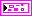Returns settings for cross validation.
H to O Coefficients Read Only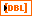Returns the trained coefficients between the hidden layer and the output layer in the trained neural network model. The number of rows equals the number of output neurons.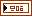If you select the Set Parameters instance of the Initialize Classification Model (NN) VI, this property returns the hyperparameters input of the VI. If you select the Search Parameters instance of the Initialize Classification Model (NN) VI, this property returns the optimal hyperparameters after Train Classification Model VI completes grid search.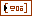Returns multiple values for each hyperparameter.Returns the method of optimization to determine the optimal hyperparameter settings.
I to H Coefficients Read OnlyReturns the trained coefficients between the input layer and the hidden layer. The number of rows equals the number of hidden neurons.
Label of Each Class Read OnlyReturns the label of each class.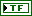Returns whether the model is initialized.Returns whether the model is trained.Returns the number of classes.
Number of Input Neurons Read OnlyReturns the number of input neurons.
Number of Output Neurons Read OnlyReturns the number of output neurons.